# What is Incidence Matrix?

Incidence matrix is that matrix which represents the graph such that with the help of that matrix we can draw a graph. This matrix can be denoted as [AC] As in every matrix, there are also rows and columns in incidence matrix [AC].
The rows of the matrix [AC] represent the number of nodes and the column of the matrix [AC] represent the number of branches in the given graph. If there are ‘n’ number of rows in a given incidence matrix, that means in a graph there are ‘n’ number of nodes. Similarly, if there are ‘m’ number of columns in that given incidence matrix, that means in that graph there are ‘m’ number of branches.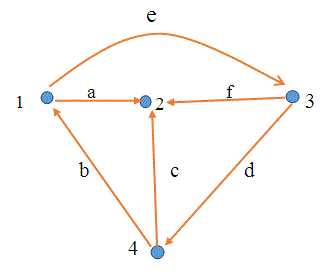In the above shown graph or directed graph, there are 4 nodes and 6 branches. Thus the incidence matrix for the above graph will have 4 rows and 6 columns.
The entries of incidence matrix is always -1, 0, +1. This matrix is always analogous to KCL (Krichoff Current Law). Thus from KCL we can derive that,

 Type of branch Value Outgoing branch from kth node +1 Incoming branch to kth node -1 Others 0

## Steps to Construct Incidence Matrix

Following are the steps to draw the incidence matrix :-

1. If a given kth node has outgoing branch, then we will write +1.
2. If a given kth node has incoming branch, then we will write -1.
3. Rest other branches will be considered 0.

## Examples of Incidence MatrixFor the graph shown above write its incidence matrix.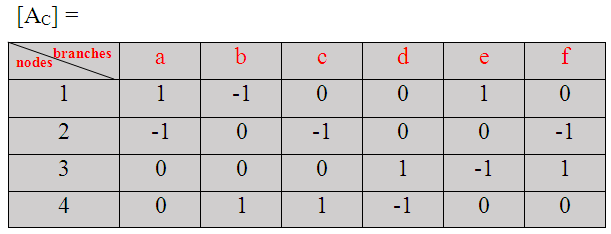## Reduced Incidence Matrix

If from a given incidence matrix [AC], any arbitrary row is deleted, then the new matrix formed will be reduced incidence matrix. It is represented by symbol [A]. The order of reduced incidence matrix is (n-1) × b where n is the number of nodes and b is the number of branches.
For the above shown graph, the reduced incidence matrix will be :-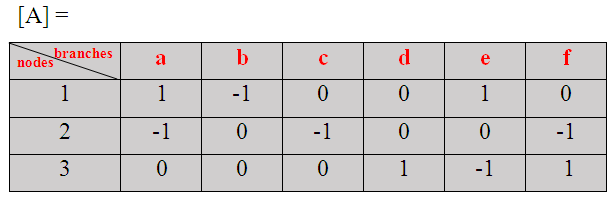[NOTE :- In the above shown matrix row 4 is deleted.]
Now let us consider a new example related to reduced incidence matrix. For the graph shown above write its reduced incidence matrix.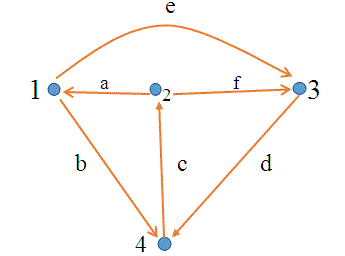Answer:- In order to draw reduced incidence matrix first of all draw its incidence matrix. Its incidence matrix is :-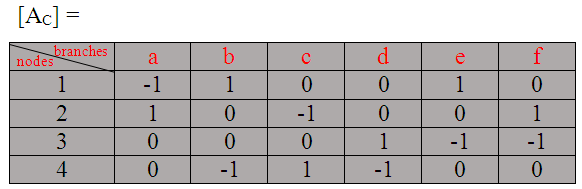Now drawing its reduced incidence matrix. For this we just simply have to delete any node (in this we have deleted node 2). Its reduced incidence matrix is:-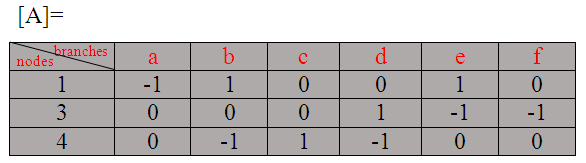Points to remember

• For checking correctness of incidence matrix which we have drawn, we should check sum of column.
• If sum of column comes to be zero, then the incidence matrix which we have created is correct else incorrect.
• The incidence matrix can be applied only to directed graph only.
• The number of entries in a row apart from zero tells us the number of branches linked to that node. This is also called as degree of that node.
• The rank of complete incidence matrix is (n-1), where n is the number of nodes of the graph.
• The order of incidence matrix is (n × b), where b is the number of branches of graph.
• From a given reduced incidence matrix we can draw complete incidence matrix by simply adding either +1, 0, or -1 on the condition that sum of each column should be zero.

Want To Learn Faster? 🎓
Get electrical articles delivered to your inbox every week.
No credit card required—it’s 100% free.